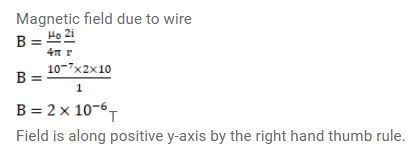# A current of 10A is established in a long wire along the positive Z-axis.

Question:

A current of $10 \mathrm{~A}$ is established in a long wire along the positive Z-axis. Find the magnetic field $\overrightarrow{\mathrm{B}}$ at the point $(1 \mathrm{~m}, 0,0)$.

Solution: